# How to solve KnapSack problem in Java using Dynamic Programming? Example Tutorial

Hello guys, if you are learning Dynamic programming and come across Knapsack problem but not able to understand it then you are not alone. It took me a lot of time to understand this problem and pattern before I can use it to solve similar kind of Dynamic Programming problems. The Knapsack problem is actually a popular computer science problem that involves selecting a set of items with the maximum possible value while keeping the weight of the selected items within a given capacity. Also, if you are new to Dynamic programming, then let me give a brief about it. Dynamic Programming is a widely used algorithmic technique to solve the Knapsack problem. In Java, we can use Dynamic Programming to efficiently solve the Knapsack problem.

If you are looking to solve the Knapsack problem in Java using Dynamic Programming, you have come to the right place. Earlier, I have shared best Dynamic programming courses and problems and In this article, we will guide you through the step-by-step process of solving the Knapsack problem in Java using Dynamic Programming.

We will start by explaining the problem statement and then provide you with a simple and easy-to-follow Java code solution that you can use to solve the Knapsack problem. Whether you are a beginner or an experienced Java developer, this guide will help you solve the Knapsack problem with ease.

Problem Statement:

You are given a KnapSack of a maximum capacity of 'W' and N items each with its own value and weight associated with it, You have to find the max value item(s) that we can put in the KnapSack of the capacity 'W'.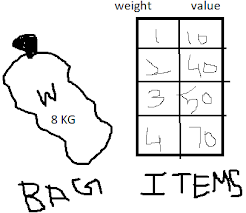KNAPSACK PROBLEM

Let's collect more input & apply the thought process?

The total weight we can put in the bag is either 8 kg or less.

But keep in mind that you can't fill an item more than one time. Repetitions are not allowed.

We are given 2 arrays, weight & value, as input data.

Finally, we need to generate/print the max value.

How to determine whether the problem can be solved using the DP(Dynamic Programming) or not?

### Let's try to solve it...

If you have noticed it is talking about the max value so we can say that it is a kind of optimization problem and we know that we can solve such a problem by using dynamic programming but it is tricky.

Consider the below matrix of knapsacks of different capacities where rows present the items to be put in the knapsack and the columns represent the knapsacks with different capacities(weights). Each cell represents the value of the given item to be put in the bag.

--------- KnapSacks with different capacities---->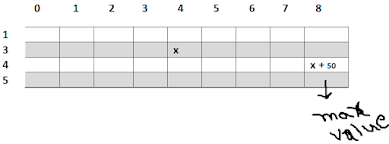We have to find the value of the last bottom right cell to get the max value for an 8 kg knapsack.

### Go In Depth...

Ask yourself why we came up with such a matrix?

In order to calculate the max value for the 8 kg KnapSack, we will have to calculate the max values of the smaller capacities KnapSacks represented as 7, 6, 5, 4, and so on.

Now let's see how it works.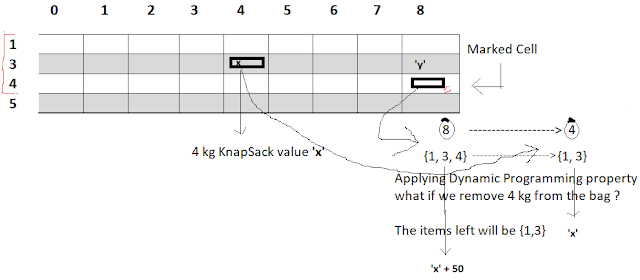For the marked cell 'matrix' we will generate the max value using dynamic programming property.

The diagram says that the values that can be put in this cell are 1, 3, and 4.

After applying the dynamic prog. property and remove the 4 kg weight item from the marked cell.

So my problem reduces to 4 kg KnapSack with items 1 and 3. Let's say we already calculated the max value 'x' for this KnapSack.

We can use this value 'x' to calculate the value of the marked cell. And the value will be x+50.

So what we did?

We just added the 4 kg weight item's value(50), which we removed from the bag in the previous step, back to the 8 kg KnapSack in the marked cell.

So the value of the marked cell, x+ 50, represents the value in 8 kg KnapSack using items 1, 3, and 4.

Now the question is it is mandatory to use items 1, 3, and 4 to put the max value in 8 kg KnapSack?

No, because the max value can be generated with values 1, and 3 as well, and consider that value is 'y'.

So finally the maximum value for the marked cell will be:

matrix = max(x+50, y);

for the given 8 kg KnapSack.

**So this is the core logic we will use to solve this problem.

Let's take another example

Suppose we are given a KnapSack of 7 kg with items 1, 3, 4, and 5 to find the value of the cell highlighted.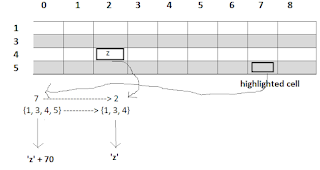Apply the same logic as the above example i.e. remove the 5 kg item.

So after removing the weight the new KnapSack comes out with a weight of 2 kg with items left are 1, 3, and 4.

Again emphasize what logic we are using ????

🤔🤔🤔

So basically whatever row we select to generate the max value of the cell, we selected the last row of the 5 kg weight item, and we remove the weight of that item from the given KnapSack as well as from the list of items.

Given KnapSack                  New KnapSack

7 ---------removing 5 ---------> 2

{1, 3, 4, 5}----removing 5 -----> {1, 3, 4}

Now let's assume that the new KnapSack generates max value 'z'. Again apply the same logic as applied in the previous example to generate the max value for the highlighted cell for 7 kg KnapSack.

The value of the removed 5 kg weight item is 70 so again adding it back to the KnapSack the value of the highlighted cell becomes 'z+70'.

Now consider that the value of the cell just above the highlighted one is 'y' then we can say that the value for the desired cell will be:

matrix = max(z+70, y);

Note: Please note that the 'y' is also generated using the same logic i.e. the max of the cells above it.

y = max(matrix, matrix);

So keep in mind that this is the basic logic to solve this problem.

Now start filling this matrix...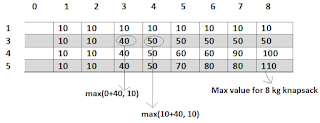For the knapsacks 1, 2, ...., 8 the max value with a given item of weight 1 kg will be 10.

Now come to the 2nd row. The max values for knapsacks 1, and 2 with given items 1 and 3 will be 10 only because the knapsack total weights 1 and 2 are less than 3 kg weight item.

Pick the value of any cell and apply the logic you will understand how the max value got generated.

But let me again explain to you for the cells 'matrix' and 'matrix'.

Given KnapSack                          Generated KnapSack

3 ----------removing 3 ---------- 0

Items Given                                 Items Left

{ 1, 3 } ---------removing 3 ------{ 1 }

The weight of the generated knapsack '0' is 0 kg so we can't put the value(10) of the item{1} left. So the max value for the new knapsack will be 0.

Now as per our logic again add removed the 3 kg weight item back to the knapsack so finally, the max value for the given knapsack 3 will be generated '0 + 40'.

Now the value above the cell 'matrix' is 10.

So matrix  = max(0 + 40, 10) = 40.

Similarly, the max value for the 8 kg knapsack will be 110.

Implementation:

Now let's covert the above problem into code.

The below logic shows how to fill the matrix above.

```for(int i = 0; i < weight.length; i++){

for(int j = 0; j <= W; j++){

matrix[i][j] = Max(matrix[i-1][j-weight[i]] + value[i] , matrix[i-1][j]);

}

}```

weight: Given an array of items weights.

value: Given an array of the item values.

The outer for loop prints the rows and the inner one prints the columns of the matrix.

The equation

` matrix[i][j] = Max(matrix[i-1][j-weight[i]] + value[i] , matrix[i-1][j]);  `

is playing the main role.

This represents the core logic that I explained to you to calculate the cell value.

Complete Code:

```public class Main  {
public static int knapSackProblem(int[] value, int[] weight, int W){
int[][] matrix = new int[value.length + 1][W + 1]

for (int i = 1; i <= value.length; i++)
{

for (int j = 0; j <= W; j++) {

if (weight[i-1] > j) {

matrix[i][j] = matrix[i-1][j];

}

else {

matrix[i][j] = Integer.max(matrix[i-1][j],matrix[i-1][j-weight[i-1]]
+ value[i-1]);

}

}

}

return matrix[value.length][W];

}

public static void main(String[] args){

int[] value = { 10,40, 50, 70 };

int[] weight = {  1,3,4,5 };

int W = 8;

System.out.println("Value is "+ knapSackProblem(value, weight, W));

}

}```

Q. 1) Given KnapSack W = 9 kg and items with different weights and corresponding values are:

 Weight value 1 80 4 70 5 90

What could be the max value after putting the items in the bag so that the total weight <= 9 kg?

Ans. 160

### Before you Leave...

Knowledge of data structure and algorithms is must to simulate the real world problem in code.